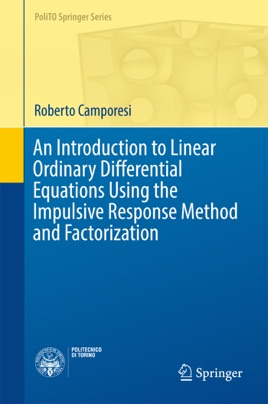• USD 84.99

## Descripción de editorial

This book presents a method for solving linear ordinary differential equations based on the factorization of the differential operator. The approach for the case of constant coefficients is elementary, and only requires a basic knowledge of calculus and linear algebra. In particular, the book avoids the use of distribution theory, as well as the other more advanced approaches: Laplace transform, linear systems, the general theory of linear equations with variable coefficients and variation of parameters. The case of variable coefficients is addressed using Mammana’s result for the factorization of a real linear ordinary differential operator into a product of first-order (complex) factors, as well as a recent generalization of this result to the case of complex-valued coefficients.